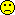## TIE for NTIDs [Power / Sample Size]

Dear Astea!

❝ And what about the different CI for two metrics? Can you invent smthg like Power2.RSABE or Power2.NTIDFDA or it is a stupid idea?

If time allows. But pensioners never have time.

From theory the intersection-union principle should protect us from alpha-inflation, as long as the single tests to be combined also have size 0.05. Since this isn't the case for RSABE for NTIDs, as Helmut has shown in his post, an alpha inflation has to be supposed.
Thus power2.NTIDFDA(), or whatever it would be called, only had the purpose to show with numbers that the TIE is not <=0.05, as ist should be. Educational purpose only. Duno if all the effort and labour to implement sumfink like power2.NTIDFDA() is worth that aim.

❝ For an extreme example, suppose we calculate sample size, basing on CV 25% (I understand that NTID should not have large variance but nevertherless): sampleN.TOST(CV=0.25, theta0=0.975, theta1=0.9, theta2=1.11, design="2x2")

❝ 122 volunteers!

This sample size estimation is for the EMA recommendation to deal with NTIDs, namely to shrinken the BE acceptance range to 0.9 ... 1.1111...

The FDA reference scabled ABE method uses the conventional limits 0.8 ... 1.25 from an CV ~ 0.214 on and higher. Below that the acceptance ranges are tightened, at swR=0.1 f.i. they are 0.9 ... 1.1111...
This leads of course to different sample sizes. Use sampleN.NTIDFDA() instead.

Moreover, the FDA method requires a replicate design in which both CVwT and CVwR are estimable. That is a full replicate "2x2x4" or a 3-period replicate "2x2x3" with sequences TRT | RTR. Although the latter is not mentioned in the Warfarine guidance.
Thus your design used in the sample size estimation, "2x2", is not possible.

❝ Then by using Power.2TOST for CV=0.3 I get TIE very slightly upper than 0.05

 power.2TOST(CV=c(0.3,0.25), n=122, theta1=c(0.8, 0.9), theta2=c(1.25, 1.11), theta0=c(1, 1.11), rho=0)

❝  0.05000002

What you here calculate is not the TIE. Finding the TIE having more than one test is much more complicated.
There are other values for theta0 which also belong to the Null "bioinequivalence", f.i. theta0 = c(0.8, 1) or theta0 = c(1.25, 1) and so on and so on. Over all these sets the maximum (supremum) of power has to be determined to get the TIE.
This is implemented in the function type1error.2TOST().
Be warned: The run-time of this function in the development version, after changing to simulations as basic concept, is horrible. Some minutes are the normal case.

Hope this doesn't cause headache to you. Two or more TOSTs is for hardcore statisticians only. And they also have difficulties as I know (I'm not a statistician).

Regards,

DetlewIng. Helmut Schütz